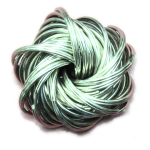# Bordism and Cobordism

Monday, July 23rd, 2012 | Author:Two connected compact manifolds N and M are said to be bordant, if there exists a manifold W with boundary consisting of two connected components isomorphic to N and M respectively. The name comes from french and means sharing a boundary. Some people say cobordant, since the manifolds don't share a boundary but "are" shared as a boundary (I don't know how to explain this better than with the definition given above). We will stick to "bordant" because we investigate precisely what "the bordism of a manifold" and "the cobordism of a manifold" are.

One can see that being bordant is an equivalence relation, so it makes sense to speak of bordism classes of manifolds. By enriching N and M with extra structure (like a tangential framing, or an orientation), we get several different notions of bordism classes.

From each of these bordism theories, we get a sequence of spaces $\Omega_n$ such that $\Omega_n$ is the Thom space of a universal bundle over some classifying space (I will explain that later) and $\Sigma \Omega_n$ is homotopy equivalent to $\Omega_{n+1}$. Homotopy theorists like to call such a sequence then a spectrum and by standard theory one can associate to each spectrum a generalized homology theory and a generalized cohomology theory. Even better, Brown's representability theorem states that every generalized (co)homology theory comes from a spectrum, so we have a 1:1 correspondence.

The goal of this article is now to define Thom spectra and to give a geometric interpretation of the corresponding homology and cohomology theories, essentially by carrying out the Pontryagin-Thom construction relatively.

Category: English, Mathematics | 2 Comments

# Thom spaces

Wednesday, June 13th, 2012 | Author:I want to discuss the elementary basics of Thom spaces of vector bundles. To start, I explain general one-point compactification and a different construction on vector spaces, then I do it for vector bundles to define the Thom space. I also discuss suspension of topological spaces and how adding a trivial vector space (or bundle) corresponds to suspension under the forming of Thom spaces.

Motto: (Thom space:Suspension)::(Vector bundle:Trivial bundle) or

"Thom spaces are twisted suspensions"

Honestly, I really want to talk about algebraic (motivic) Thom spaces some day, but these are some preliminaries to understand what's going on, so I want to get this out first.

Category: English, Mathematics | 2 Comments